The product of two numbers is 120 and the sum of their squares is 289. The sum of the number is.

# The product of two numbers is 120 and the sum of their squares is 289. The sum of the number is.

1. A
15
2. B
23
3. C
18
4. D
17

Fill Out the Form for Expert Academic Guidance!l

+91

Live ClassesBooksTest SeriesSelf Learning

Verify OTP Code (required)

### Solution:

Let the number be x and y.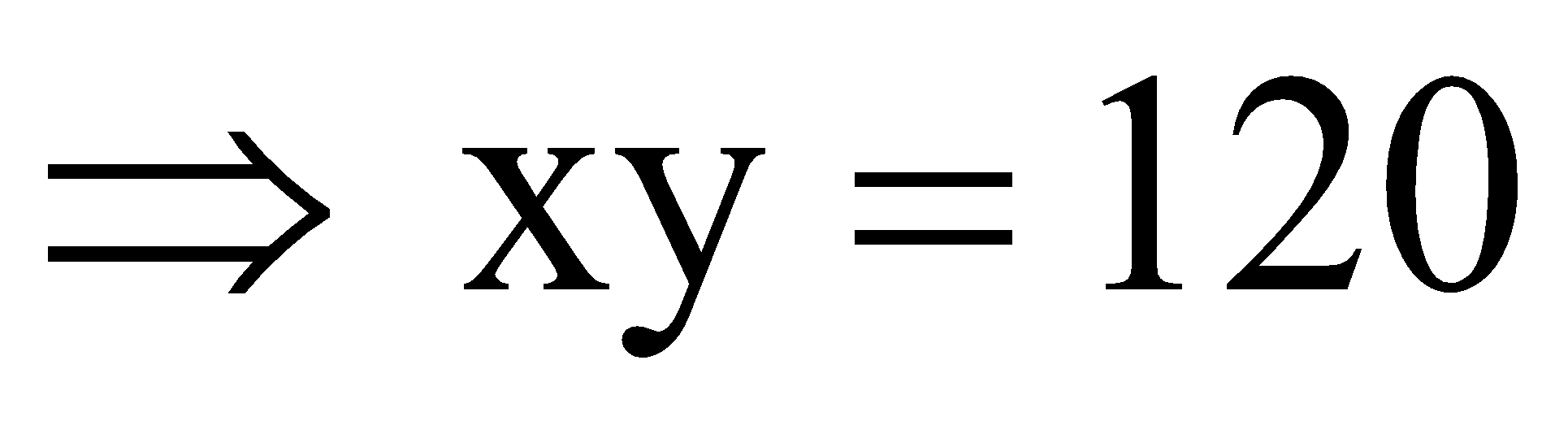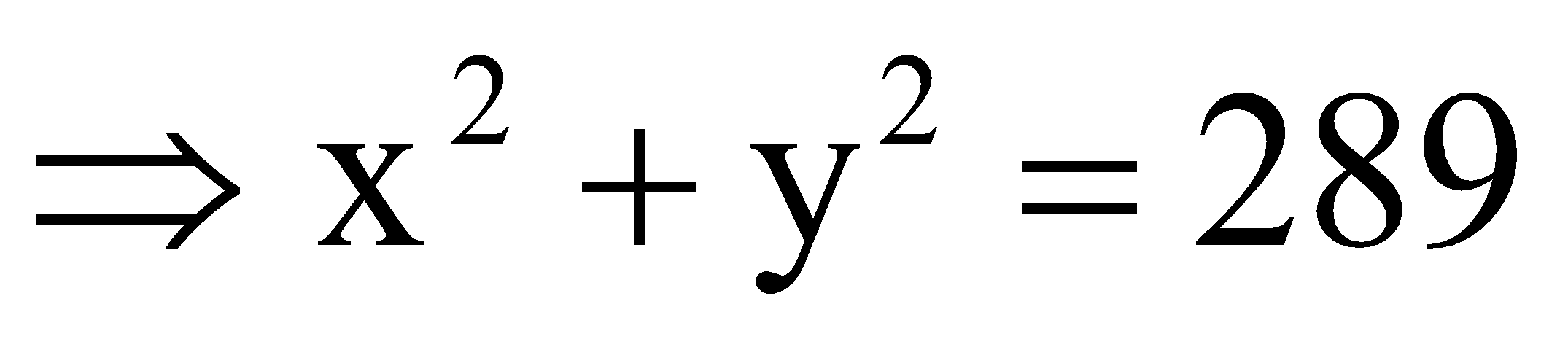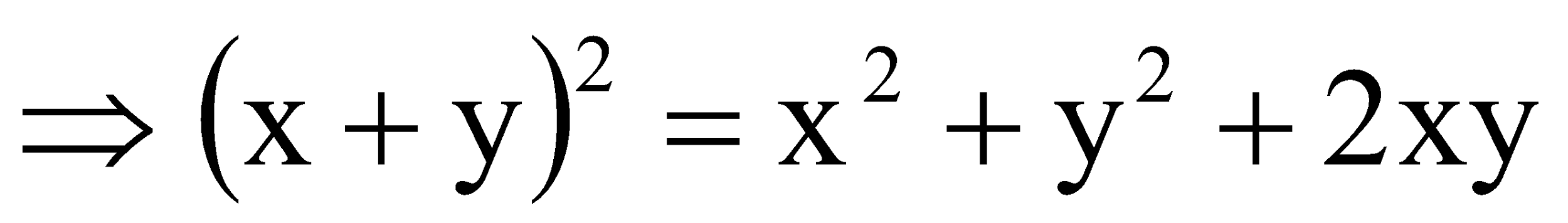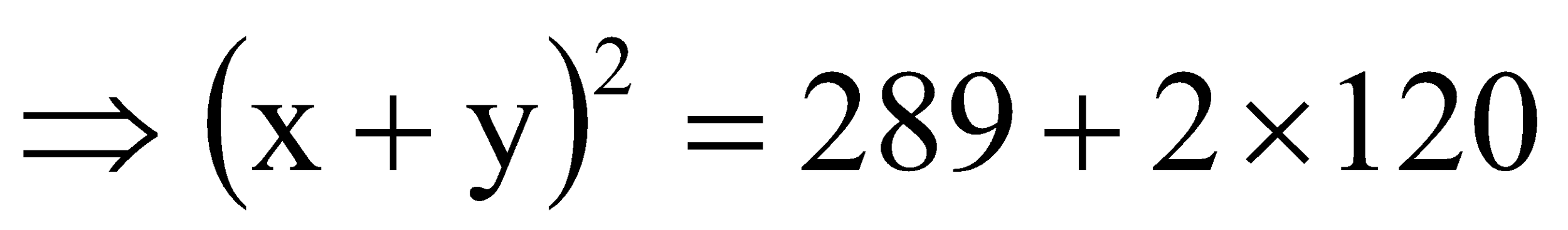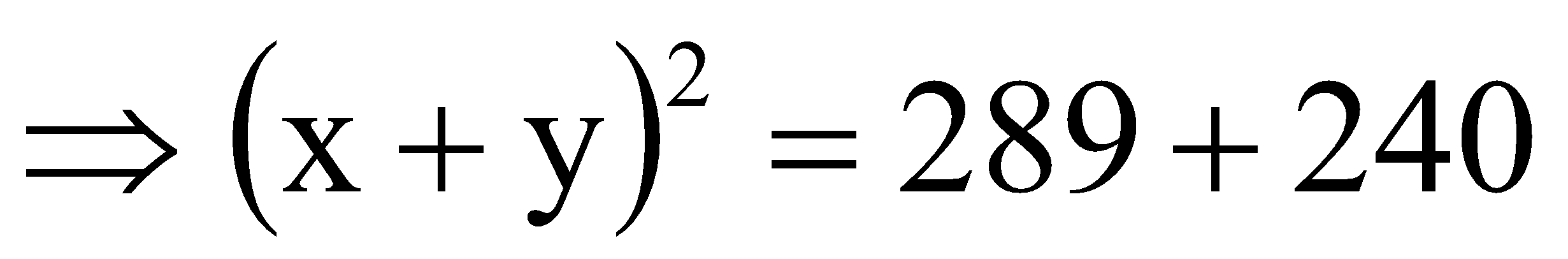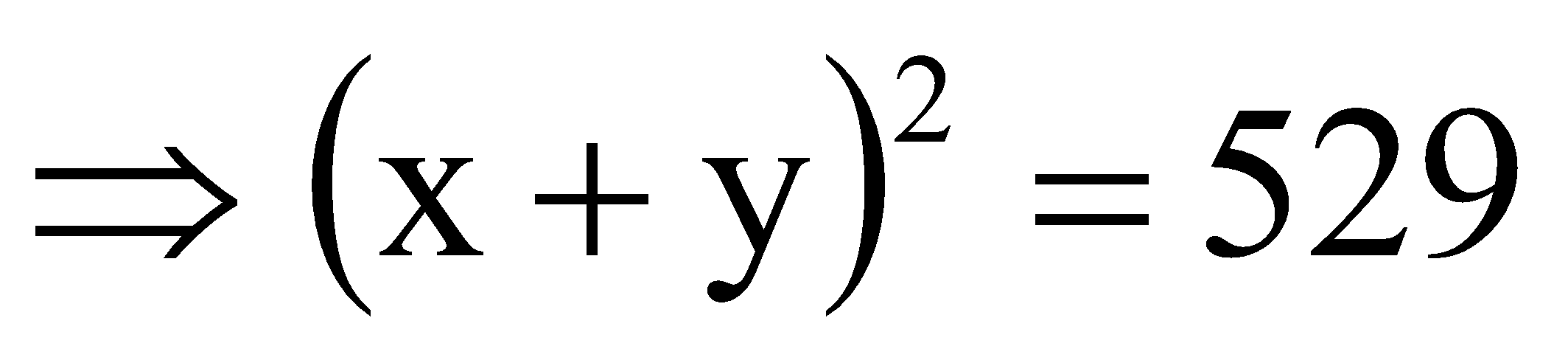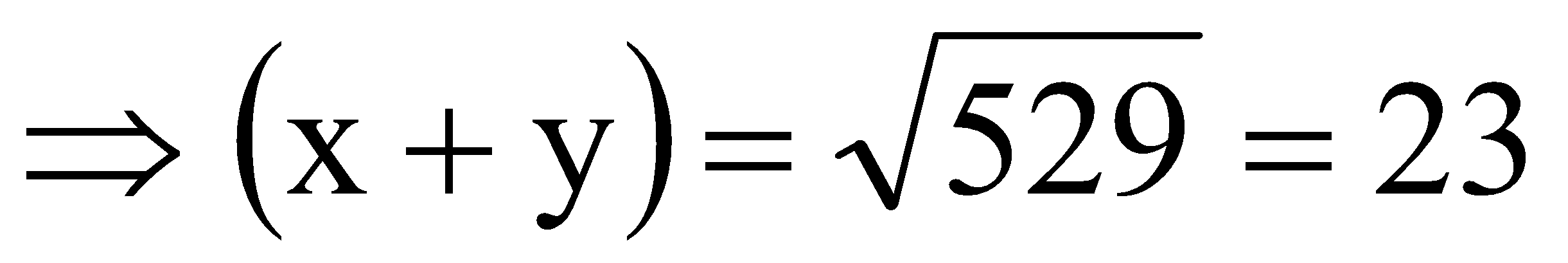.

## Related content

 Area of Square Area of Isosceles Triangle Pythagoras Theorem Triangle Formula Perimeter of Triangle Formula Area Formulae Volume of Cone Formula Matrices and Determinants_mathematics Critical Points Solved Examples Type of relations_mathematics+91

Live ClassesBooksTest SeriesSelf Learning

Verify OTP Code (required)